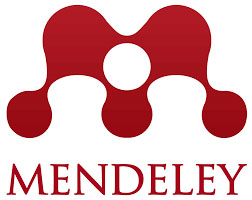rscosan.com

# Paper: Symmetry of terminating series representations of the Askey-Wilson polynomials

 ACADEMIC PRESS INC ELSEVIER SCIENCE (USA) | ISSN: 0022-247X | JCI® 2022 Impact Factor: 1.417 - MATHEMATICS — position: 77/333 (Q1/T1) title## Symmetry of terminating series representations of the Askey-Wilson polynomials

Cohl, H. S. and Costas-Santos, R. S. Journal of Mathematical analysis and applications 517, no. 1 (2023), 15 pages

## Abstract

In this paper, we explore the symmetric nature of the terminating basic hypergeometric series representations of the Askey-Wilson polynomials and the corresponding terminating basic hypergeometric transformations that these polynomials satisfy. In particular we identify and classify the set of 4 and 7 equivalence classes of terminating balanced $${}_4\phi_3$$ and terminating very-well poised $${}_8W_7$$ basic hypergeometric series which are connected with the Askey-Wilson polynomials. We study the inversion properties of these equivalence classes and also identify the connection of both sets of equivalence classes with the symmetric group $$S_6$$, the symmetry group of the terminating balanced $${}_4\phi_3$$. We then use terminating balanced $${}_4\phi_3$$ and terminating very-well poised $${}_8W_7$$ transformations to give a broader interpretation of Watson’s $$q$$-analog of Whipple’s theorem and its converse. We give a broad description of the symmetry structure of the terminating basic hypergeometric series representations of the Askey-Wilson polynomials.

353 KB Preprint (PDF, 17 Pages, 5 Tables, 2 Figures)

## BibTeX

@article {MR4474848,
AUTHOR={Cohl, Howard S. and Costas-Santos, Roberto S.},
TITLE={Symmetry of terminating basic hypergeometric series representations of the {A}skey-{W}ilson polynomials},
JOURNAL={J. Math. Anal. Appl.},
FJOURNAL={Journal of Mathematical Analysis and Applications},
VOLUME={517},
YEAR={2023},
NUMBER={1},
PAGES={Paper No. 126583, 15},
ISSN={0022-247X},
MRCLASS={33D15},
MRNUMBER={4474848},
DOI={10.1016/j.jmaa.2022.126583},
URL={https://doi.org/10.1016/j.jmaa.2022.126583},
}